Foxtable(狐表)用户栏目专家坐堂 → 新建行后自动选中

共有409人关注过本帖树形打印复制链接

# 主题：新建行后自动选中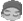nuoyan88
1楼 | 信息 | 搜索 | 邮箱 | 主页 | UC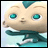加好友发短信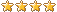老师，我选中了一行后把选中的这行的内容复制到新的一行中，我想让新的一种是自动选中的，因为新增后还要手动点击下才能打勾。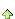2楼 | 信息 | 搜索 | 邮箱 | 主页 | UC加好友发短信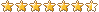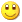Post By：2019/9/15 21:16:00 [只看该作者]

 复制后，给新行变量勾选不就行了For Each dr1 As DataRow In DataTables("表A").Select("[金额] > 100")    Dim dr2 As Row = Tables("表B").AddNew()    dr2.checked = tureNextnuoyan88
3楼 | 信息 | 搜索 | 邮箱 | 主页 | UC加好友发短信Post By：2019/9/15 21:23:00 [只看该作者]

 框起来的地方新增后直接是打勾的，现在是没有打勾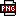此主题相关图片如下：1.png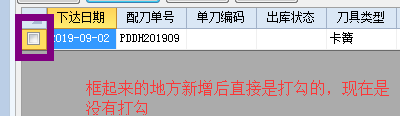4楼 | 信息 | 搜索 | 邮箱 | 主页 | UC加好友发短信Post By：2019/9/15 21:25:00 [只看该作者]

 新增不可能会自动打勾的，都需要自己写代码勾nuoyan88
5楼 | 信息 | 搜索 | 邮箱 | 主页 | UC加好友发短信Post By：2019/9/15 21:57:00 [只看该作者]

 如果按照以下代码那样，就出现了如图的，新增有内容的没有选中，没有内容的却自动选中了此主题相关图片如下：1.png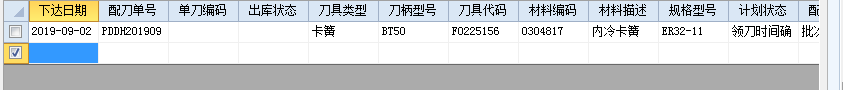Dim t As Table = Forms("刀具归还明细").Controls("计划明细").TableFor Each r As Row In Tables("刀具归还明细_计划明细").GetCheckedRows    Dim r2 As Row = t.AddNew    r2("使用设备") =r("使用设备")    r2("加工工序") = r("加工工序")    r2("刀柄型号") = r("刀柄型号")    r2("加工内容") = r("加工内容")    r2("规格型号") = r("规格型号")    r2("刀具类型") = r("刀具类型")    r2("刀具代码") = r("刀具代码")    r2("成品编码") = r("成品编码")    r2("成品描述") = r("成品描述")    r2("毛坯产地") = r("毛坯产地")    r2("材料编码") = r("材料编码")    r2("材料描述") = r("材料描述")    r2("配刀类型") = r("配刀类型")    r2("数量") = r("数量")    r2("加工次数") = r("加工次数")    r2("下达日期") = r("下达日期")    r2("生产批次") = r("生产批次")    r2("线别") = r("线别")    r2("配刀单号") = r("配刀单号")    r2("计划状态") = "已完成"    r2("计划用刀日期") = Date.Today()    r2("备注") = r("备注")NextTables("刀具归还明细_计划明细").position = Tables("刀具归还明细_计划明细").rows.count - 1Tables("刀具归还明细_计划明细").HideUnSelectedRowsDim dr2 As Row = Tables("刀具归还明细_计划明细").AddNew()dr2.checked = True6楼 | 信息 | 搜索 | 邮箱 | 主页 | UC加好友发短信Post By：2019/9/15 22:44:00 [只看该作者]

 Dim t As Table = Forms("刀具归还明细").Controls("计划明细").TableFor Each r As Row In Tables("刀具归还明细_计划明细").GetCheckedRows    Dim r2 As Row = t.AddNewr2..checked = Truenuoyan88
7楼 | 信息 | 搜索 | 邮箱 | 主页 | UC加好友发短信Post By：2019/9/19 19:36:00 [只看该作者]

 老师，红色部分的代码加进去之后如图1圈起来的地方还是不能自动打勾。请帮忙看看，谢谢   Dim t As Table = Forms("刀具归还明细").Controls("计划明细").TableFor Each r As Row In Tables("刀具归还明细_计划明细").GetCheckedRows    Dim r2 As Row = t.AddNew    r2.checked = True    r2("使用设备") =r("使用设备")    r2("加工工序") = r("加工工序")    r2("刀柄型号") = r("刀柄型号")    r2("加工内容") = r("加工内容")    r2("规格型号") = r("规格型号")    r2("刀具类型") = r("刀具类型")    r2("刀具代码") = r("刀具代码")    r2("成品编码") = r("成品编码")    r2("成品描述") = r("成品描述")    r2("毛坯产地") = r("毛坯产地")    r2("材料编码") = r("材料编码")    r2("材料描述") = r("材料描述")    r2("配刀类型") = r("配刀类型")    r2("数量") = r("数量")    r2("加工次数") = r("加工次数")    r2("下达日期") = r("下达日期")    r2("生产批次") = r("生产批次")    r2("配刀业务") = "日常换刀"    r2("线别") = r("线别")    r2("配刀单号") = r("配刀单号")    r2("计划状态") = "已完成"    r2("计划用刀日期") = Date.Today()    r2("备注") = r("备注")NextTables("刀具归还明细_计划明细").position = Tables("刀具归还明细_计划明细").rows.count - 1Tables("刀具归还明细_计划明细").HideUnSelectedRowsDim t1 As Table = Forms("刀具归还明细").Controls("计划明细").TableFor Each r As Row In Tables("刀具归还明细_计划明细").GetCheckedRows    Dim r2 As Row = t1.AddNew    r2.checked = TrueNextForms("单刀配置").Open此主题相关图片如下：1.png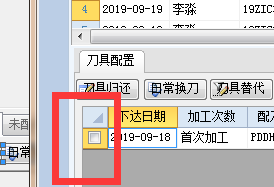8楼 | 信息 | 搜索 | 邮箱 | 主页 | UC加好友发短信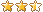Post By：2019/9/19 22:51:00 [只看该作者]

 从头看到尾，都不知道你问的是什么。nuoyan88
9楼 | 信息 | 搜索 | 邮箱 | 主页 | UC加好友发短信Post By：2019/9/20 8:09:00 [只看该作者]

 标注红色前面的代码是新建复制内容的然后把别的行隐藏，现在想达到的目的是我想这条新建的行能自行选中，也就是7楼那样框起来的地方是打勾的，但现在不是的。我查了下原因，导致不能选中的就是以下这两句代码。如果想达到我要的结果怎么把这两种代码综合呢？谢谢       Tables("刀具归还明细_计划明细").position = Tables("刀具归还明细_计划明细").rows.count - 1Tables("刀具归还明细_计划明细").HideUnSelectedRows [此贴子已经被作者于2019/9/20 8:22:27编辑过]10楼 | 信息 | 搜索 | 邮箱 | 主页 | UC加好友发短信Post By：2019/9/20 8:52:00 [只看该作者]

 去掉Tables("刀具归还明细_计划明细").HideUnSelectedRows红色代码改为Dim t1 As Table = Forms("刀具归还明细").Controls("计划明细").TableFor Each r As Row In t1.Rows    If r.Checked = False Then r.RemoveNext总数 12 1 2 下一页